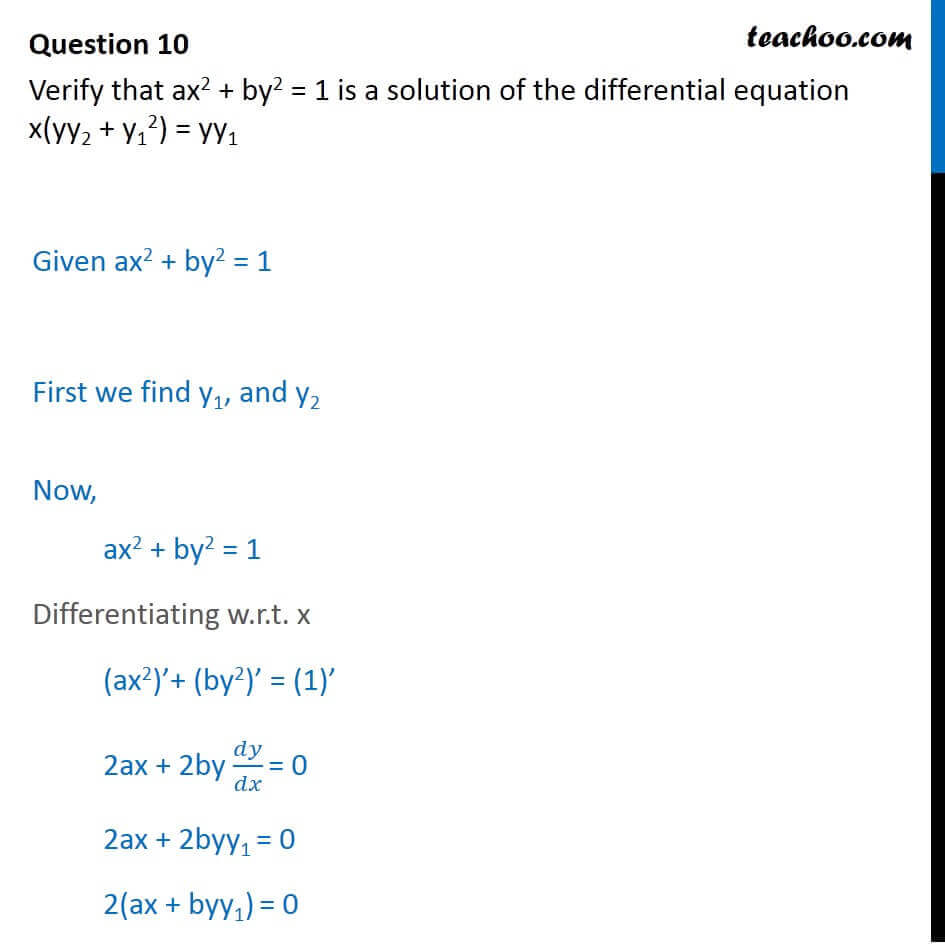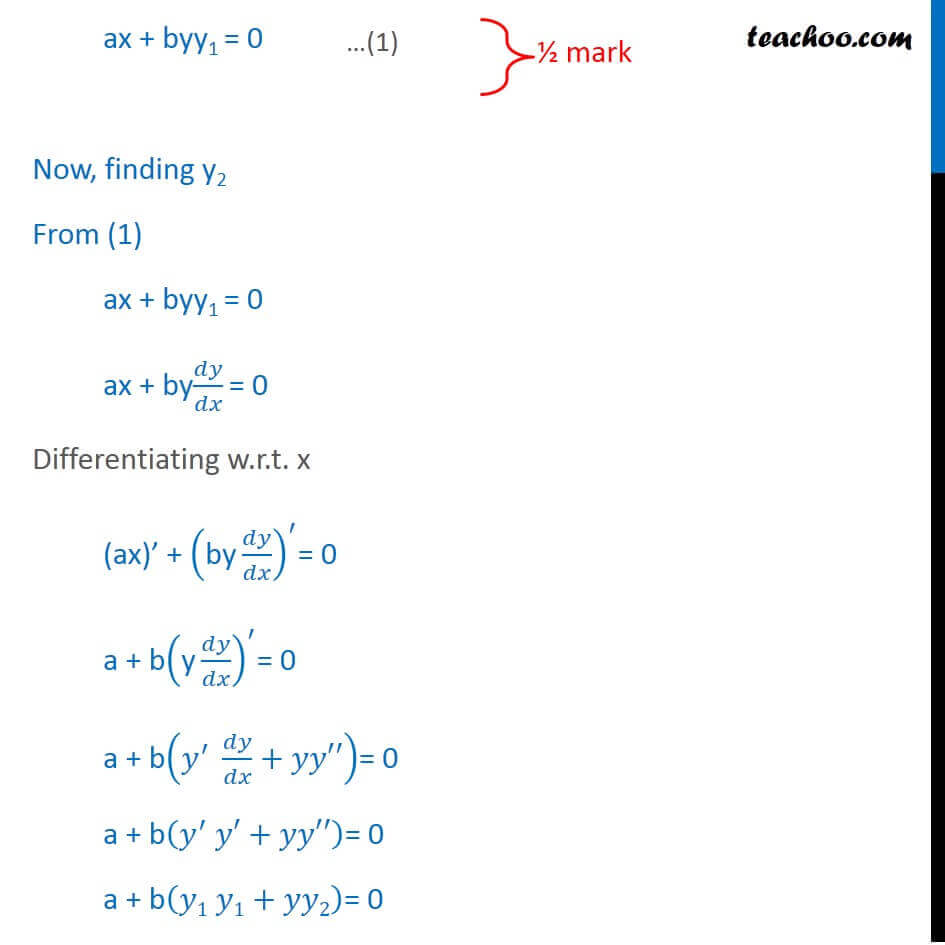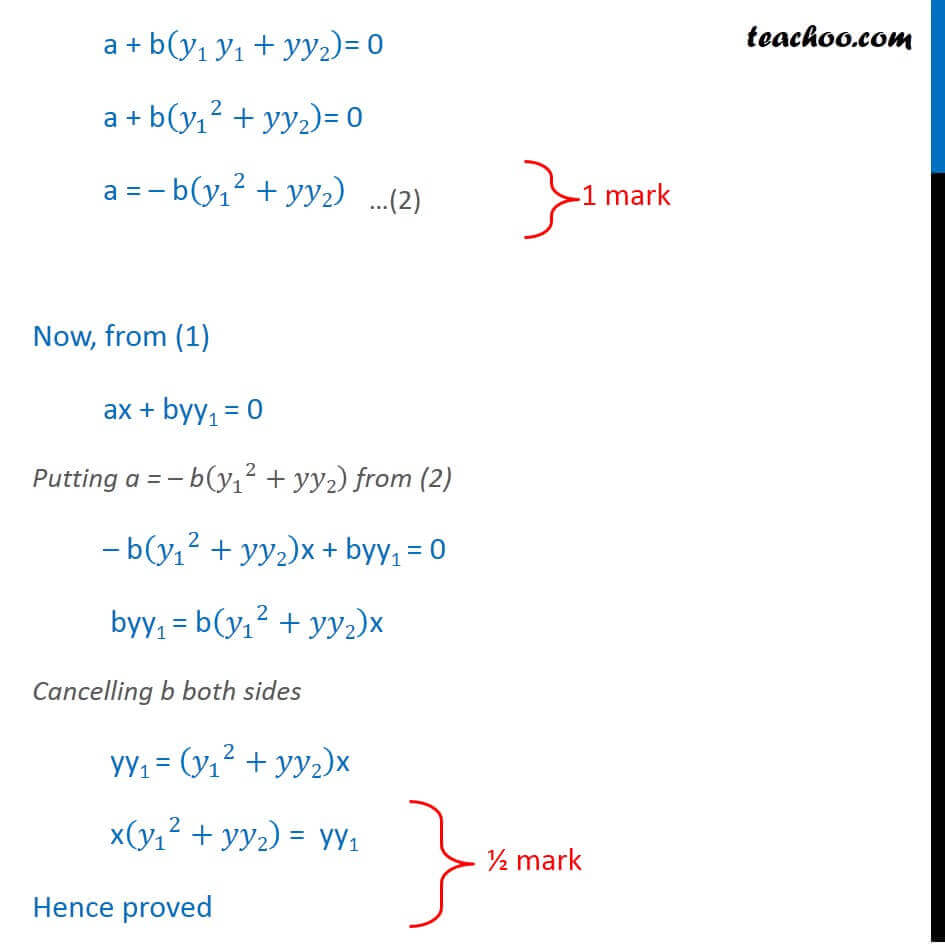CBSE Class 12 Sample Paper for 2018 Boards

Class 12
Solutions of Sample Papers and Past Year Papers - for Class 12 Boards

### Verify that ax 2 + by 2 = 1 is a solution of the differential equation x(yy 2 + y 1 2 ) = yy 1

This is a question of CBSE Sample Paper - Class 12 - 2017/18.Learn in your speed, with individual attention - Teachoo Maths 1-on-1 Class

### Transcript

Question 10 Verify that ax2 + by2 = 1 is a solution of the differential equation x(yy2 + y12) = yy1 Given ax2 + by2 = 1 First we find y1, and y2 Now, ax2 + by2 = 1 Differentiating w.r.t. x (ax2)’+ (by2)’ = (1)’ 2ax + 2by 𝑑𝑦/𝑑𝑥 = 0 2ax + 2byy1 = 0 2(ax + byy1) = 0 ax + byy1 = 0 Now, finding y2 From (1) ax + byy1 = 0 ax + by𝑑𝑦/𝑑𝑥 = 0 Differentiating w.r.t. x (ax)’ + ("by" 𝑑𝑦/𝑑𝑥)^′= 0 a + b("y" 𝑑𝑦/𝑑𝑥)^′= 0 a + b(𝑦^′ 𝑑𝑦/𝑑𝑥+𝑦𝑦′′)= 0 a + b(𝑦^′ 𝑦′+𝑦𝑦′′)= 0 a + b(𝑦1 𝑦1+𝑦𝑦2)= 0 a + b(𝑦1 𝑦1+𝑦𝑦2)= 0 a + b(〖𝑦_1〗^2+𝑦𝑦2)= 0 a = – b(〖𝑦_1〗^2+𝑦𝑦2) Now, from (1) ax + byy1 = 0 Putting a = – b(〖𝑦_1〗^2+𝑦𝑦2) from (2) – b(〖𝑦_1〗^2+𝑦𝑦2)x + byy1 = 0 byy1 = b(〖𝑦_1〗^2+𝑦𝑦2)x Cancelling b both sides yy1 = (〖𝑦_1〗^2+𝑦𝑦2)x x(〖𝑦_1〗^2+𝑦𝑦2) = yy1 Hence proved